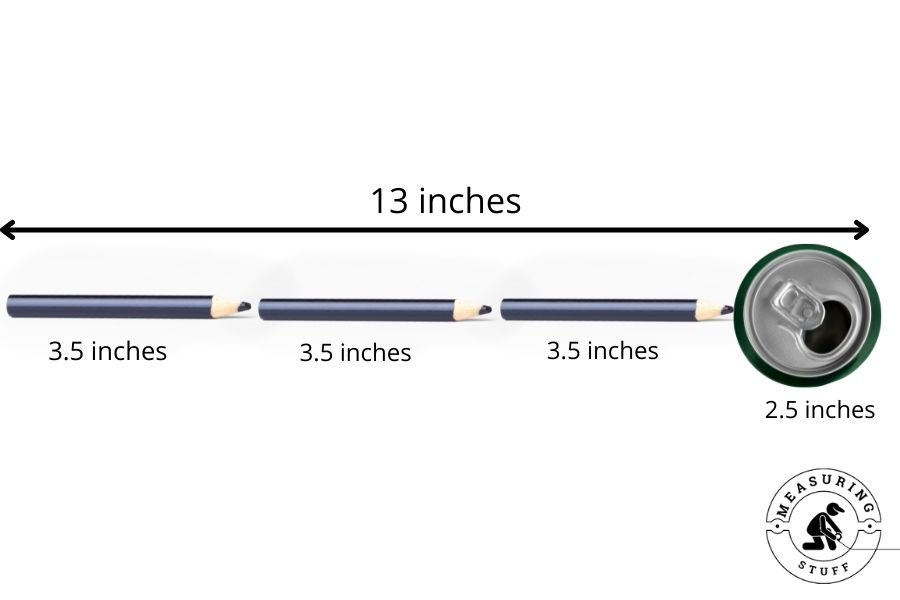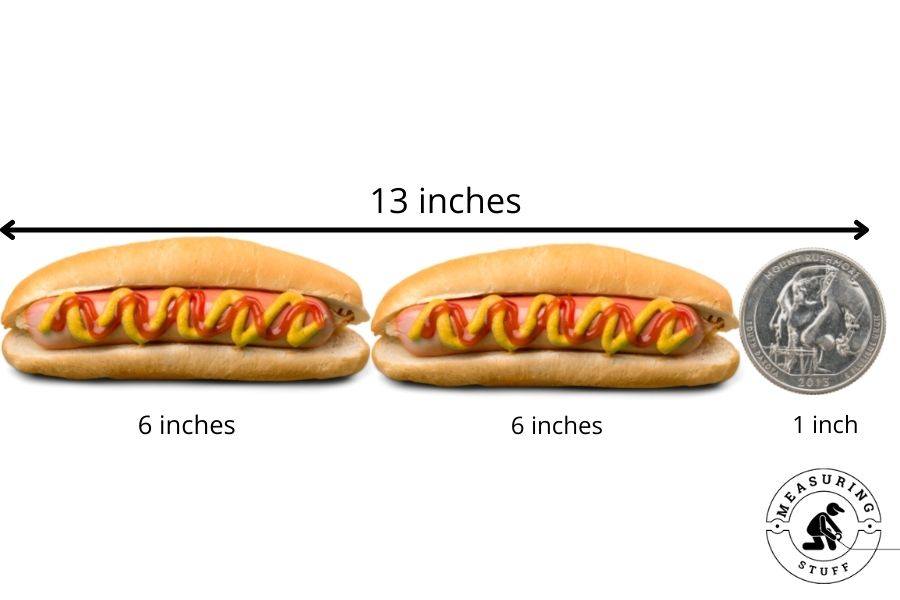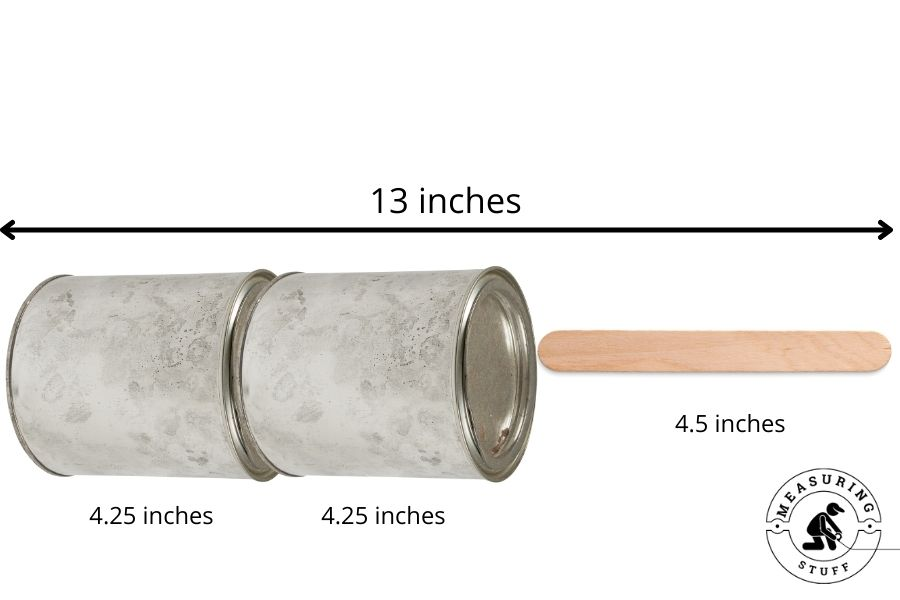# How long Is 13 Inches? (With Examples)

13 inches is a short length that can easily be measured with a ruler or measuring tape.

But without a measuring tool, knowing exactly how long 13 inches is can be difficult.

In this case, one of the best methods to use for measuring something is to compare items of similar length that you are familiar with.

This article will show you exactly how long 13 inches is with examples of items that equal 13 inches in length.

## Convert 13 inches to other measurements

13 inches is equal to 1 inch larger than a foot. It is also equal to 33.02 centimeters and 330.2 millimeters.

Refer to the following chart to convert 13 inches into other units of measurements.

## Conversion calculator

You can also use our online conversion calculator to easily convert 13 inches to other units of measurements.

Simply type in the desired value, in this case, 13, and select inches in the convert from box and desired measurement in the convert to box.

The calculator will instantly do the math for you.

## Items that are 13 inches long

13 Bottlecaps

The common plastic bottlecap that you would see on a soda bottle is great to use when referencing length.

Bottlecaps are easy to use as they are readily available and people are familiar with their size.

Each bottlecap is 1 inch in diameter. So if you placed 13 of these together in a row, they would equal 13 inches long and give you a great example of how long 13 inches is.

### Macbook Pro display

If you are an Apple user and have a Macbook Pro, you can use the diagonal dimensions of the screen display to reference something that is 13 inches long.

Although it is not exactly 13 inches (13.3), it is close enough to give you a sense of how long 13 inches is.

### 2 Pens + 1 paperclip

Common items that are used around the house or office make great tools for measuring.

For example, a standard pen is close to 6 inches long. Placing two pens together will equal 12 inches long. The common size for a paperclip is 1 inch long.

Therefore placing 2 pens (12 inches) and 1 paperclip (1 inch) together in a row will equal 13 inches long.2 pens + 1 paperclip = 13 inches long

### 3 Crayons + 1 soda can

A typical crayon that is used for coloring and drawing, is 3.5 inches long.

If you measure the length of 3 of them, they will equal 10.5 inches long.

A 12-ounce soda can measures close to 2.5 inches in diameter across the top of the can.

Therefore 3 crayons (10.5 inches) and 1 soda can (2.5 inches) placed together will equal very close to 13 inches long.3 crayons + 1 soda can = 13 inches long

### 2 Hot dogs + 1 quarter

Not all hot dogs are the same size. They can vary in length with some of them measuring 1 foot long.

But on average, a regular sized hot dog will be 6 inches long. So if you had 2 hot dogs together, they would equal 12 inches long.

In the USA, a 25 cent or quarter coin, is 1 inch in diameter. The quarter is a great item to use when looking to measure something that is 1 inch long.

Knowing that 2 hot dogs (12 inches) and 1 USA quarter (1 inch) equal 13 inches long will give you a visual reference of how long 13 inches really is.2 hot dogs + 1 quarter = 13 inches

### 2 Soup cans + 1 popsicle stick

A regular sized can of soup will be 4.25 inches tall. Therefore 2 of these cans will equal 8.5 inches long.

A popsicle stick is around 4.5 inches long (4.475 to be exact).

If you combine 2 soup cans (8.5 inches) and 1 popsicle stick (4.5 inches) together, they will equal close to 13 inches long.2 soup cans + 1 popsicle stick = 13 inches long

## Related articles:

Sharing is caring!